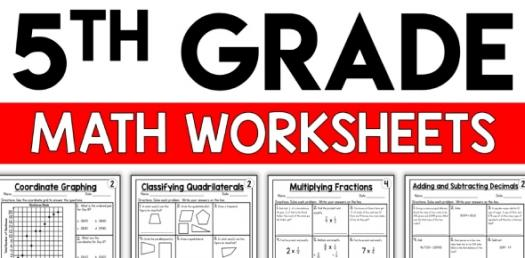# 5th Grade Math Trivia On Table Test! Quiz

20 Questions | Total Attempts: 355Settings5th grade math trivia on table test! One of the ways that people get to learn the basics of multiplication is by knowing how the multiplication table is designed and most people do so by reciting it over and over. To help see just how good you are when it comes to this table, we have prepared the quiz below, how about you check it out and see how good your memory is. .

• 1.
8x12=[Blank]
• 2.
7x7=[Blank]
• 3.
9x14=[Blank]
• 4.
6x14=[Blank]
• 5.
8x6=[Blank]
• 6.
7x15=[Blank]
• 7.
6x12=[Blank]
• 8.
9x13=[Blank]
• 9.
3X12=[Blank]
• 10.
10x1000=[Blank]
• 11.
11x90=[Blank]
• 12.
12x9=[Blank]
• 13.
13x5=
• 14.
8x8=[Blank]
• 15.
7x6=[Blank]
• 16.
15x2=[Blank]
• 17.
6x8=[Blank]
• 18.
14x7=[Blank]
• 19.
7x13=[Blank]
• 20.
9x15=[Blank]
Related TopicsBack to top